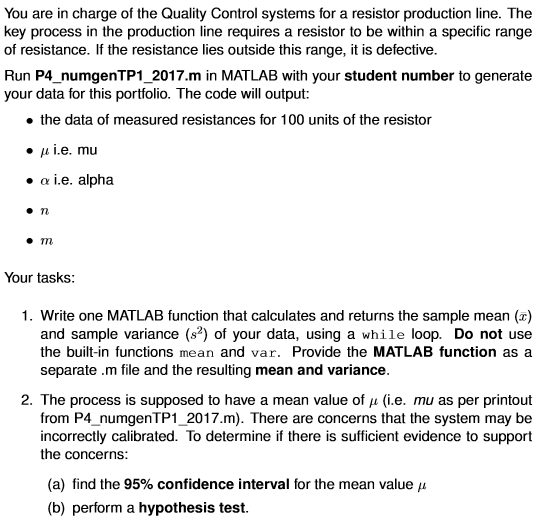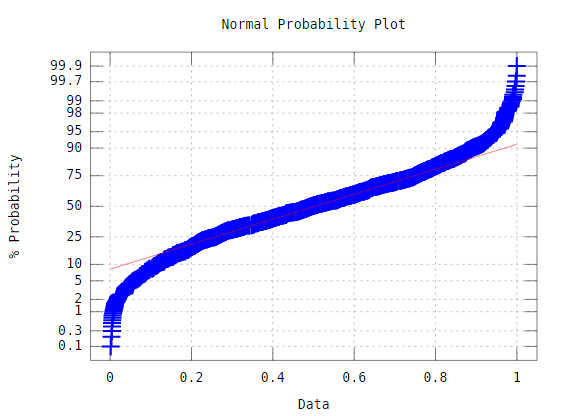# (Solved Homework): You are in charge of the Quality Control systems for a resistor production line. The key process in the production linefunction V=P4_numgenTP1_2017(9801081)

randn(‘seed’,98.0108);

rand(‘seed’,108.9009);

A1=[50,100,200,500,1000,2000,5000];
A=A1(randi(7));

B=(randi(15)+5)/400;

%V=(randn(1,50)*(rand()*20+5)/300+(1+(randi(2)*2-3)*(rand()+0.2)/100)^2)*A;
V=(randn(1,100)*(rand()*20+5)/300+(1+(randi(2)*2-3)*(rand()+0.2)/100)^2)*A;

fprintf(‘nmu=%gnnalpha=%gnnn=%gnnm=%gnn’,A,A*B,20+round(rand()*1000)/100,35+round(rand()*1000)/100);

end

You are in charge of the Quality Control systems for a resistor production line. The key process in the production line requires a resistor to be within a specific range of resistance. If the resistance lies outside this range, it is defective Run P4 numgenTP1 2017. m in MATLAB with your student number to generate your data for this portfolio. The code will output: the data of measured resistances for 100 units of the resistor Au i.e. mul a e. alpha Your tasks: 1. Write one MATLAB function that calculates and returns the sample mean (T) and sample variance (s?) of your data, using a while loop. Do not use the built-in functions mean and var. Provide the MATLAB function as a separate .m file and the resulting mean and variance. 2. The process is supposed to have a mean value of w (i.e. mu as per printout from P4 numgenTP1 2017.m). There are concerns that the system may be incorrectly calibrated. To determine if there is sufficient evidence to support the concerns: (a) find the 95% confidence interval for the mean value Au (b) perform a hypothesis test.

1)

function [_mean, variance] = findMeanVar(A)

_sum=0;
for i=1:length(A)
_sum=_sum+A(i);
end
_mean=_sum/length(A); %mean
var_sum=0;
for i=1:length(A)
var_sum=var_sum+ (A(i)-_mean)^2;
end
variance = var_sum/length(A); %Varaince
end
_mean = 0;
variance = 0;
[_mean variance] = findMeanVar(A);
fprintf(‘mean: %f’,_mean)
fprintf(‘nVariance: %f’,variance)

Sample output

```mean: 0.489680
Variance: 0.081566
```

—————————————————————————————————————————————————————

2)

a)

function [_mean, variance] = findMeanVar(A)

_sum=0;
for i=1:length(A)
_sum=_sum+A(i);
end
_mean=_sum/length(A); %mean
var_sum=0;
for i=1:length(A)
var_sum=var_sum+ (A(i)-_mean)^2;
end
variance = var_sum/length(A); %Varaince
end
_mean = 0;
variance = 0;
[_mean variance] = findMeanVar(A);
fprintf(‘mean: %f’,_mean)
fprintf(‘nVariance: %f’,variance)

standard_error = std(A)/sqrt(length(A)); % Standard Error
total_score = tinv([0.025 0.975],length(A)-1); % T-Score
Confidence_interval = _mean + total_score*standard_error; % Confidence Intervals
fprintf(‘nConfidence interval: %f’,Confidence_interval)

Sample output

```mean: 0.495790
Variance: 0.082837
Confidence interval: 0.477921
Confidence interval: 0.513660
```

—————————————————————————————————————————————————————-

2)b)

function [_mean, variance] = findMeanVar(A)

_sum=0;
for i=1:length(A)
_sum=_sum+A(i);
end
_mean=_sum/length(A); %mean
var_sum=0;
for i=1:length(A)
var_sum=var_sum+ (A(i)-_mean)^2;
end
variance = var_sum/length(A); %Varaince
end
_mean = 0;
variance = 0;
[_mean variance] = findMeanVar(A);
fprintf(‘mean: %f’,_mean)
fprintf(‘nVariance: %f’,variance)

standard_error = std(A)/sqrt(length(A)); % Standard Error
total_score = tinv([0.025 0.975],length(A)-1); % T-Score
Confidence_interval = _mean + total_score*standard_error; % Confidence Intervals
fprintf(‘nConfidence interval: %f’,Confidence_interval)
normplot(A)

Sample outputOrder NOW for a 10% Discount
Pages (550 words)
Approximate price: -

Why Us?Top Quality and Well-Researched Papers

All ourbpapers are written from scratch. In fact, Clients who ask for paraphrasing services are highly discouraged. We have writers ready to craft any paper from scratch and deliver quality ahead of time.Our writers keeps you posted on your papers progress - providing you with paper outline/draft. You are also at liberty to communicate directly with your writer.Free Unlimited Revisions

If you think we missed something, send your order for a free revision. You have 10 days to submit the order for review after you have received the final document. You can do this yourself after logging into your personal account or by contacting our support.Prompt Delivery and 100% Money-Back-Guarantee

All papers are always delivered on time, in many cases quite ahead of time. In case we need more time to master your paper, we may contact you regarding the deadline extension. In case you cannot provide us with more time, a 100% refund is guaranteed.Original & Confidential

We use several writing tools checks to ensure that all documents you receive are free from plagiarism. Our editors carefully review all quotations in the text. We also promise maximum confidentiality in all of our services.Our support agents are available 24 hours a day 7 days a week and committed to providing you with the best customer experience. Get in touch whenever you need any assistance.

Try it now!

## Calculate the price of your order

Total price:
\$0.00

How it works?Fill in the order form and provide all details of your assignment.Proceed with the payment

Choose the payment system that suits you most.Our Services

No need to work on your paper at night. Sleep tight, we will cover your back. We offer all kinds of writing services.## Essay Writing Service

No matter what kind of academic paper you need and how urgent you need it, you are welcome to choose your academic level and the type of your paper at an affordable price. We take care of all your paper needs and give a 24/7 customer care support system.An admission essay is an essay or other written statement by a candidate, often a potential student enrolling in a college, university, or graduate school. You can be rest assurred that through our service we will write the best admission essay for you.Editing Support

Our academic writers and editors make the necessary changes to your paper so that it is polished. We also format your document by correctly quoting the sources and creating reference lists in the formats APA, Harvard, MLA, Chicago / Turabian.Revision Support

If you think your paper could be improved, you can request a review. In this case, your paper will be checked by the writer or assigned to an editor. You can use this option as many times as you see fit. This is free because we want you to be completely satisfied with the service offered.